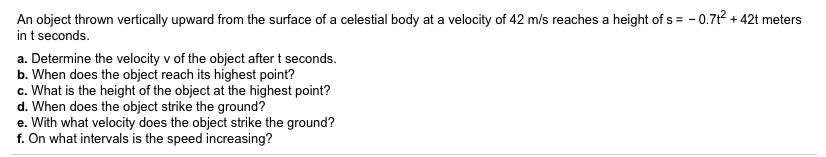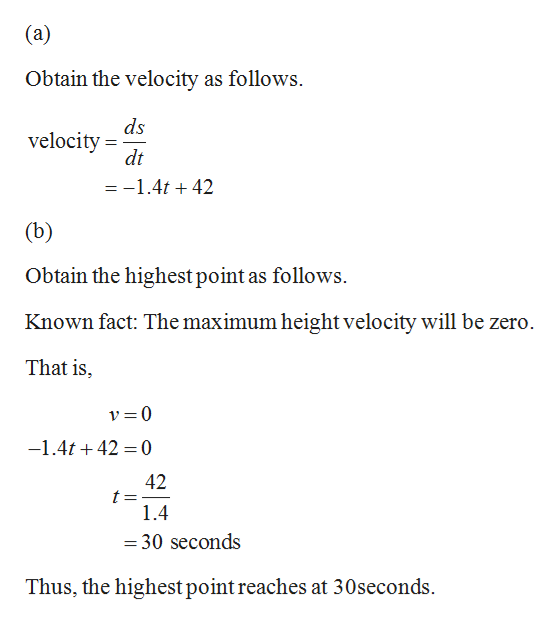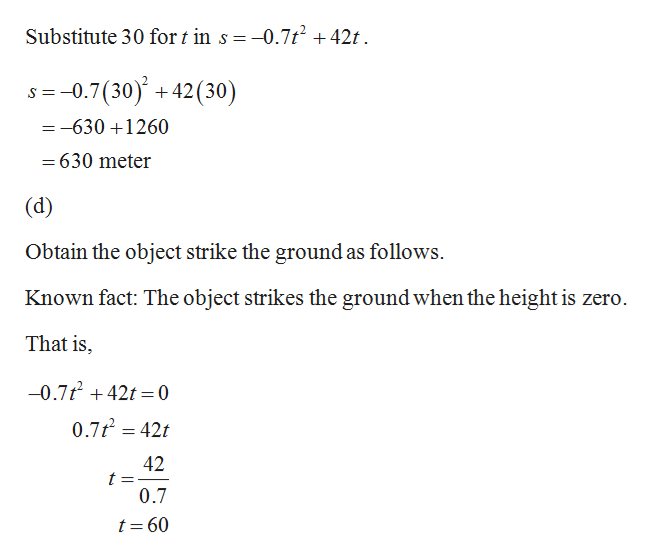# -0.7t2+42t metersAn object thrown vertically upward from the surface of a celestial body at a velocity of 42 m/s reaches a height of sin t secondsa. Determine the velocity v of the object after t seconds.b. When does the object reach its highest point?c. What is the height of the object at the highest point?d. When does the object strike the ground?e. With what velocity does the object strike the ground?f. On what intervals is the speed increasing?

Question
22 viewshelp_outlineImage Transcriptionclose-0.7t2+42t meters An object thrown vertically upward from the surface of a celestial body at a velocity of 42 m/s reaches a height of s in t seconds a. Determine the velocity v of the object after t seconds. b. When does the object reach its highest point? c. What is the height of the object at the highest point? d. When does the object strike the ground? e. With what velocity does the object strike the ground? f. On what intervals is the speed increasing? fullscreen
check_circle

Step 1

From the given statement, the height of the object is s = – 0.7t2 + 42t meters in t seconds.help_outlineImage Transcriptionclose(a) Obtain the velocity as follows ds velocity dt =-1.4t 42 (b) Obtain the highest point as follows. Known fact: The maximum height velocity will be zero That is v=0 -1.4t42 0 42 1.4 = 30 seconds Thus, the highest point reaches at 30seconds fullscreen
Step 2

(c)

Obtain the height of the high...help_outlineImage TranscriptioncloseSubstitute 30 for t in s = -0.7t2 +42t S 0.7(30)42(30) --630 1260 =630 meter (d) Obtain the object strike the ground as follows Known fact: The object strikes the ground when the height is zero. That is -0.7t420 0.7t 42t 42 t = 0.7 t=60 fullscreen

### Want to see the full answer?

See Solution

#### Want to see this answer and more?

Solutions are written by subject experts who are available 24/7. Questions are typically answered within 1 hour.*

See Solution
*Response times may vary by subject and question.
Tagged in

### Other